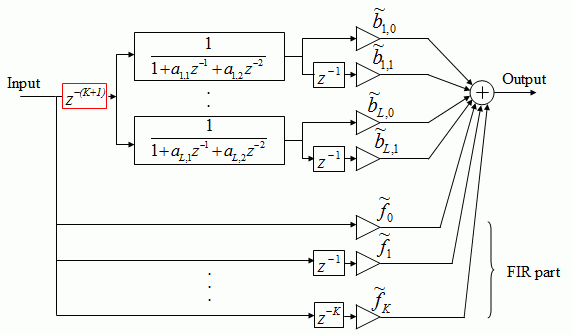# A delayed parallel filter structure with an FIR part with improved numerical propertiesThis is the companion page of the paper
Balázs Bank and Julius O. Smith, III, "A delayed parallel filter structure with an FIR part having improved numerical properties", 136th AES Convention, Preprint No. 9084, Berlin, April 2014.

##Abstract

In real-world applications high-order IIR filters are often converted to series or parallel second-order sections to decrease the negative effects of coefficient truncation and roundoff noise. While series biquads are more common, the parallel structure is gaining more interest due to the possibility of full code parallelization. In addition, it is relatively simple to design a filter directly in a parallel form, which can be efficiently utilized for logarithmic frequency resolution filtering often required in audio. If the numerator order of the original transfer function is higher than that of the denominator, a parallel FIR part arises in addition to the second-order IIR sections. Unfortunately, in this case the gain of the sections and that of the FIR filter can be significantly higher than that of the final transfer function, which requires the downscaling of the filter coefficients to avoid overload. This leads to a significant loss of useful bitdeph. This paper analyzes this problem and suggests delaying the IIR part so that there is no overlap between the responses of the FIR part and the second-order sections.Fig. 1: the structure of the delayed parallel filter.

##MATLAB code

Here you can find Matlab functions for obtaining delayed parallel second-order filters, which should also work in Octave (all files as a zip file):

• delparfilt - Filtering operation using the delayed parallel filter structure

Here are the commands required for convering a direct-form B(z)/A(z) filter to the delayed parallel form:

• residued - Alternate partial fraction expansion with delayed partial fractions (details here)
• rpk2parf - Converts the partial fraction expansion form to parallel second-order sections
• tf2delparf - Does the direct form -> delayed parallel form conversion in one step
• tf2delparf_example - Example script and comparison with the non-delayed parallel filter

Next, let's see what can we do with fixed-pole parallel filters (more about them here):

And there are some auxiliary files needed for the comparison:

• parfilt - Filtering operation using the normal (non-delayed) parallel filter structure
• tf2parf - Converts the B(z)/A(z) transfer function to normal (non-delayed) parallel form
• parfiltdes - Original time-domain LS design of the fixed-pole parallel second-order filter
• freqpoles - Calculating the poles of the parallel filter based on a predetermined (e.g. logarithmic) pole frequency set
• fe167.mat - The measured loudspeaker impulse response used in the example scripts
• tfplot - Logarithmic transfer-function plot for displaying the results

You can find more info about fixed-pole parallel filters here and you may also want to check my entire list of publications.

(c) Balazs Bank, 2014. All rights of the codes reserved to the author. Non-commercial use permitted, for all other uses, please contact the author.